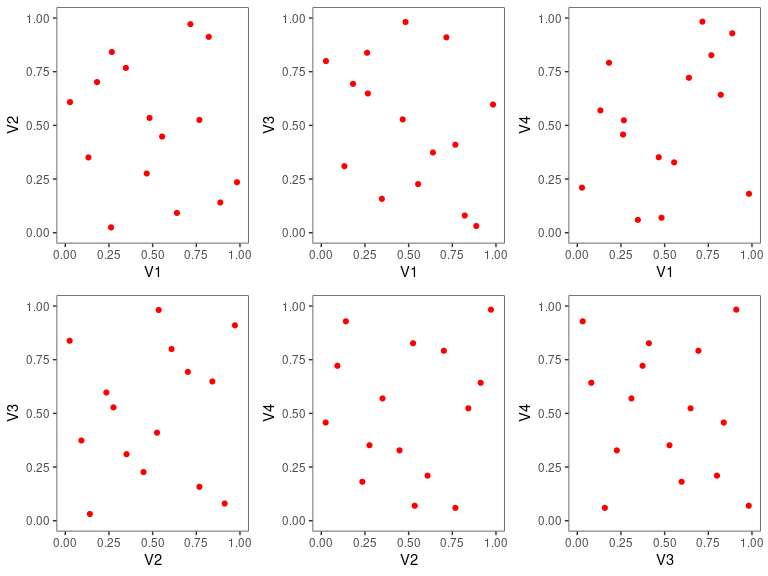# Overview of ggDoE

The ggDoE package provides an easy approach to creating commonly used graphs in Design of Experiments with the R package ggplot2. The following plots are currently available in ggDoE:

1. Alias Matrix
2. Box-Cox Transformation
3. Lambda Plot
4. Boxplots
5. Regression Diagnostic Plots
6. Half-Normal Plot
7. Interaction Effects Plot for a Factorial Design
8. Main Effects Plot for a Factorial Design
9. Contour Plots for Response Surface Methodology
10. Pareto Plot
11. Two Dimensional Projections of a Latin Hypercube Design

The following datasets/designs are included in ggDoE as tibbles:

“Experiments: Planning, Analysis, and Optimization, 2nd Edition”
ggDoE::adapted_epitaxial
#> # A tibble: 16 × 7
#>        A     B     C     D  ybar    s2  lns2
#>    <dbl> <dbl> <dbl> <dbl> <dbl> <dbl> <dbl>
#>  1    -1    -1    -1     1  14.6 0.270 -1.31
#>  2    -1    -1    -1    -1  13.6 0.291 -1.23
#>  3    -1    -1     1     1  14.2 0.268 -1.32
#>  4    -1    -1     1    -1  14.0 0.197 -1.62
#>  5    -1     1    -1     1  14.6 0.221 -1.51
#>  6    -1     1    -1    -1  13.9 0.205 -1.59
#>  7    -1     1     1     1  14.4 0.222 -1.51
#>  8    -1     1     1    -1  14.1 0.215 -1.54
#>  9     1    -1    -1     1  14.7 0.269 -1.31
#> 10     1    -1    -1    -1  13.7 0.272 -1.30
#> 11     1    -1     1     1  13.8 0.220 -1.51
#> 12     1    -1     1    -1  13.9 0.229 -1.47
#> 13     1     1    -1     1  14.6 0.227 -1.48
#> 14     1     1    -1    -1  13.9 0.253 -1.37
#> 15     1     1     1     1  14.3 0.250 -1.39
#> 16     1     1     1    -1  14.1 0.192 -1.65
• original_epitaxial: Original epitaxial layer experiment obtain from the book
“Experiments: Planning, Analysis, and Optimization, 2nd Edition”
ggDoE::original_epitaxial
#> # A tibble: 16 × 7
#>        A     B     C     D  ybar       s2  lns2
#>    <dbl> <dbl> <dbl> <dbl> <dbl>    <dbl> <dbl>
#>  1    -1    -1    -1     1  14.8 0.00312  -5.77
#>  2    -1    -1    -1    -1  13.9 0.00494  -5.31
#>  3    -1    -1     1     1  14.8 0.00333  -5.70
#>  4    -1    -1     1    -1  13.9 0.000926 -6.98
#>  5    -1     1    -1     1  14.9 0.00269  -5.92
#>  6    -1     1    -1    -1  14.2 0.00415  -5.48
#>  7    -1     1     1     1  14.9 0.0165   -4.11
#>  8    -1     1     1    -1  14.0 0.00196  -6.24
#>  9     1    -1    -1     1  14.9 0.215    -1.54
#> 10     1    -1    -1    -1  14.0 0.121    -2.12
#> 11     1    -1     1     1  14.4 0.206    -1.58
#> 12     1    -1     1    -1  13.9 0.226    -1.49
#> 13     1     1    -1     1  14.9 0.147    -1.92
#> 14     1     1    -1    -1  14.0 0.0880   -2.43
#> 15     1     1     1     1  14.8 0.327    -1.12
#> 16     1     1     1    -1  13.9 0.0704   -2.65
• aliased_design: D-efficient minimal aliasing design obtained from the article
“Efficient Designs With Minimal Aliasing”
ggDoE::aliased_design
#> # A tibble: 12 × 5
#>        A     B     C     D     E
#>    <dbl> <dbl> <dbl> <dbl> <dbl>
#>  1    -1     1    -1     1     1
#>  2    -1     1     1     1    -1
#>  3    -1    -1     1    -1     1
#>  4     1     1     1     1     1
#>  5     1     1     1    -1    -1
#>  6     1    -1    -1     1    -1
#>  7    -1     1    -1    -1     1
#>  8     1    -1     1     1     1
#>  9     1     1    -1    -1    -1
#> 10    -1    -1     1    -1    -1
#> 11     1    -1    -1    -1     1
#> 12    -1    -1    -1     1    -1
library(ggDoE)

## Alias Matrix

Let $$X_1$$ denote the model matrix for the main-effects model (including the intercept) and let $$X_2$$ denote the model matrix corresponding to the two-factor interactions.

Suppose the true model is $Y = X_1 \beta_1 + X_2 \beta_2 + \epsilon$ where $$\epsilon$$ is the vector of residuals with $$\mathbb{E}(\epsilon)=0$$, $$\beta_1$$ is the vector consisting of the intercept and main effects coefficients and $$\beta_2$$ is the vector consisting of the two-factor interactions effects coefficients.

If the experimenter employs the main effects model for estimation, it is well known that the expected value of the least squares estimator $$\hat{\beta}_1$$ of $$\beta_1$$ is

$\mathbb{E}(\hat{\beta}_1) = \beta_1 + A \beta_2$ where $$A$$ is the alias matrix.

We define the alias matrix $$A$$ by $A = (X_1^T \, X_1)^{-1} \ X_1^T X_2$

One can view the color map of the absolute value of the correlations among the main effects and two-factor interaction columns using the function alias_matrix()

alias_matrix(design=aliased_design)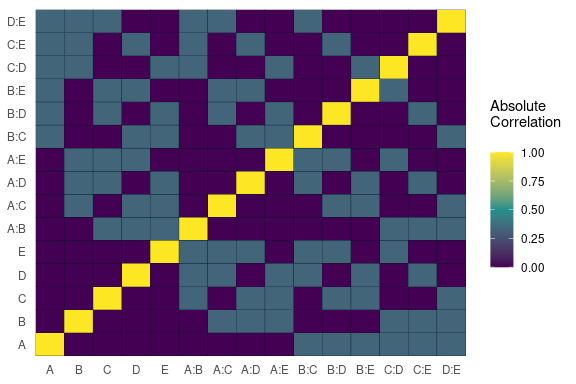For most functions in ggDoE one can choose to not display the plot and only return the data/calculations used to construct the plot by using showplot=FALSE argument. For example, we can simply return the correlation matrix (not absolute) among the main effects and two-factor interactions as follows

alias_matrix(design=aliased_design, showplot=FALSE)
#>      A:B   A:C    A:D    A:E    B:C    B:D    B:E   C:D    C:E   D:E
#> A  0.000 0.000  0.000  0.000  0.333 -0.333 -0.333 0.333  0.333 0.333
#> B  0.000 0.333 -0.333 -0.333  0.000  0.000  0.000 0.333 -0.333 0.333
#> C  0.333 0.000  0.333  0.333  0.000  0.333 -0.333 0.000  0.000 0.333
#> D -0.333 0.333  0.000  0.333  0.333  0.000  0.333 0.000  0.333 0.000
#> E -0.333 0.333  0.333  0.000 -0.333  0.333  0.000 0.333  0.000 0.000

## Box-Cox Transformation

Box-Cox transformation is a transformation of a response variable that often is not normally distributed, to one that does follow approximately a normal distribution. The transformation performed is as follows

$y(\lambda) = \begin{cases} \frac{y^\lambda -1}{\lambda}, & \text{if} \, \lambda \neq 0 \\ \log y, & \text{if} \, \lambda =0 \end{cases}$

The “optimal value” of $$\lambda$$ is one which results in the best approximation of a normal distribution curve.

Some common Box-Cox transformations are

$$\lambda$$ $$y(\lambda)$$
-2 $$\frac{1}{y^2}$$
-1 $$\frac{1}{y}$$
-$$\frac{1}{2}$$ $$\frac{1}{\sqrt{y}}$$
0 $$\log y$$
$$\frac{1}{2}$$ $$\sqrt{y}$$
1 $$y$$
2 $$y^2$$
model <- lm(s2 ~ (A+B+C+D),data = adapted_epitaxial)
boxcox_transform(model,lambda = seq(-5,5,0.2))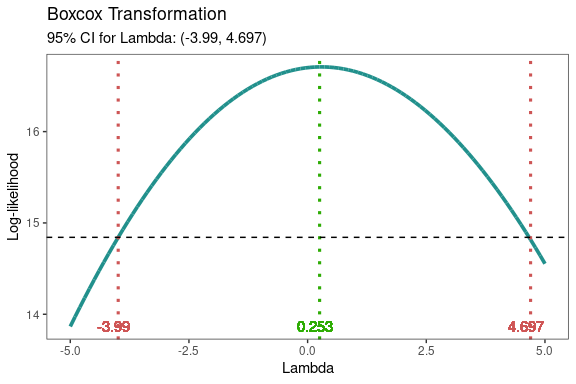From the above figure, the “optimal value” of $$\lambda=0.253$$. A good transformation to perform would then be $$\log(y)$$. The results can be extracted by using showplot=FALSE argument, if needed.

boxcox_transform(model,lambda = seq(-5,5,0.2),
showplot = FALSE)
#> # A tibble: 1 × 3
#>   best_lambda lambda_low lambda_high
#>         <dbl>      <dbl>       <dbl>
#> 1       0.253      -3.99        4.70

The transformation doesn’t always work well, so make sure to check the diagnostics of the model with the transformed response. See diagnostic_plots().

## Lambda Plot

Obtain the trace plot of the t-statistics calculated for each effect in the model after applying Box-Cox transformation across a specified sequence of lambda values

model <-  lm(s2 ~ (A+B+C)^2,data=original_epitaxial)
lambda_plot(model)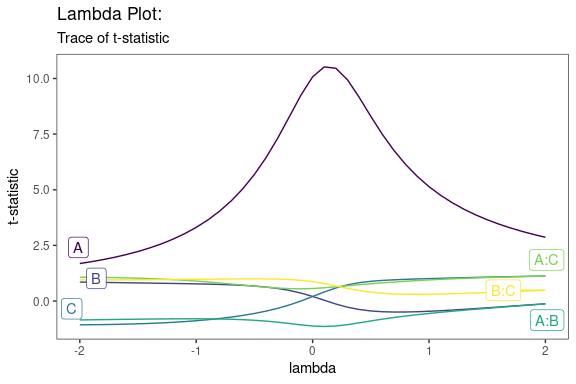or alternatively,

lambda_plot(model, showplot=FALSE)
#> # A tibble: 41 × 7
#>    lambda     A     B      C  A:B A:C B:C
#>     <dbl> <dbl> <dbl>  <dbl>  <dbl> <dbl> <dbl>
#>  1   -2    1.69 0.850 -1.07  -0.851 1.07  0.945
#>  2   -1.9  1.77 0.843 -1.07  -0.844 1.07  0.946
#>  3   -1.8  1.86 0.835 -1.06  -0.837 1.06  0.947
#>  4   -1.7  1.97 0.828 -1.05  -0.830 1.05  0.949
#>  5   -1.6  2.09 0.820 -1.04  -0.823 1.04  0.951
#>  6   -1.5  2.23 0.813 -1.02  -0.816 1.03  0.954
#>  7   -1.4  2.38 0.805 -1.01  -0.811 1.01  0.958
#>  8   -1.3  2.57 0.797 -0.984 -0.806 0.986 0.962
#>  9   -1.2  2.78 0.789 -0.957 -0.802 0.961 0.966
#> 10   -1.1  3.02 0.780 -0.925 -0.800 0.931 0.971
#> # … with 31 more rows

## Boxplots

Using gg_boxplots() constructs boxplots making it easy to visually compare the shape, the central tendency, and the variability of the samples. It is important to note the response and factor arguments for gg_boxplots() must be unquoted.

data <- ToothGrowth
data$dose <- factor(data$dose,levels = c(0.5, 1, 2),
labels = c("D0.5", "D1", "D2"))
head(data)
#>    len supp dose
#> 1  4.2   VC D0.5
#> 2 11.5   VC D0.5
#> 3  7.3   VC D0.5
#> 4  5.8   VC D0.5
#> 5  6.4   VC D0.5
#> 6 10.0   VC D0.5
gg_boxplots(data,response = 'len',
factor = 'dose')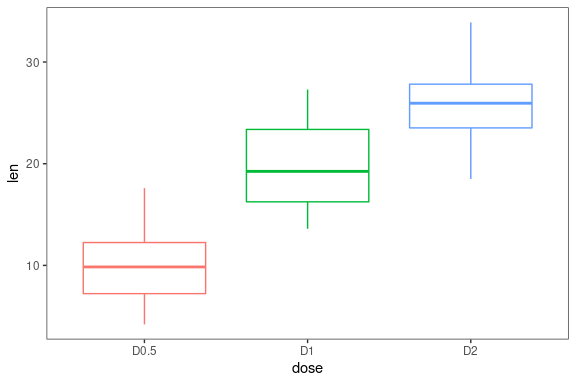We can group each sample using the group_var argument, again this argument must be unquoted. With color_palette we can change the color palette for each of the boxplots. With color_palette eight options are available: “viridis”,“cividis”,“magma”,“inferno”,“plasma”,“rocket”,“mako”,“turbo”. Lastly, one can overlay jittered points to each boxplot using jitter_points=TRUE.

gg_boxplots(data,response = 'len',
factor = 'dose',
group_var = 'supp',
color_palette = 'viridis',
jitter_points = TRUE)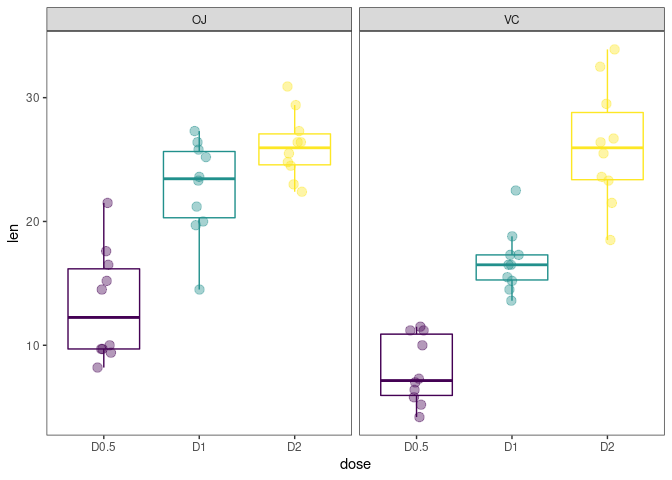## Regression Diagnostic Plots

1. Residual vs. Fitted Values
2. Normal-QQ plot
3. Scale-Location plot
4. Residual vs. Leverage
5. Cook’s Distance
6. Collinearity
model <- lm(mpg ~ wt + am + gear + vs * cyl, data = mtcars)

The default plots in diagnostic_plots() are the first four in the list mentioned above. However, one can choose any of the six plots they need. For example if one needs all six plots use which_plots=1:6.

diagnostic_plots(model, which_plots=1:6)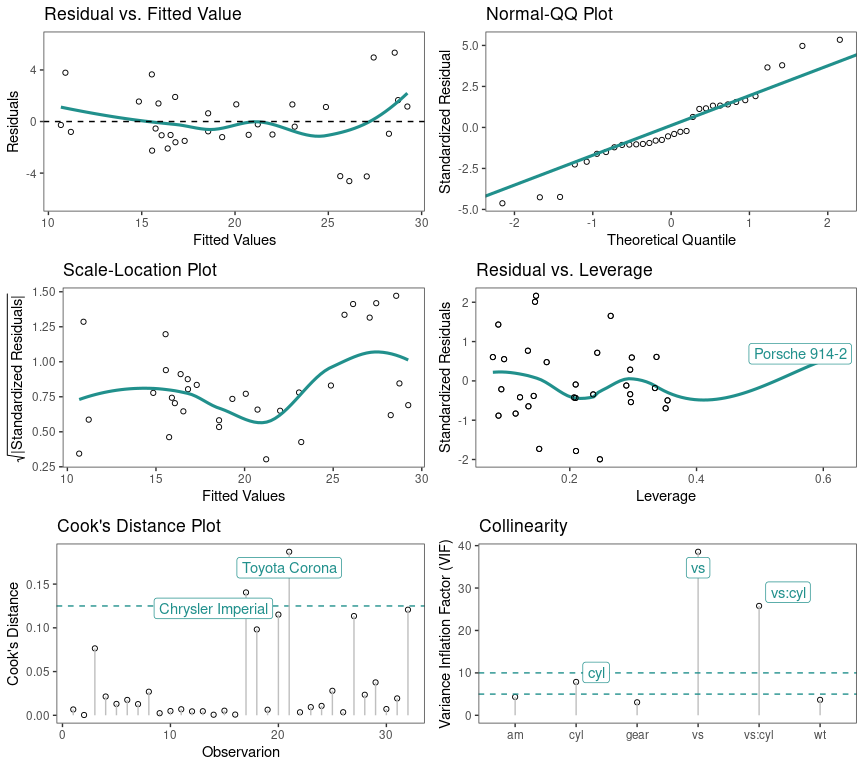Another example, if one simply requires the Residual vs. Fitted Values and Cook’s Distance plots, use which_plots = c(1,5). If one wants to display confidence intervals, use standard_error=TRUE

diagnostic_plots(model, which_plots=c(1,5),
standard_errors = TRUE)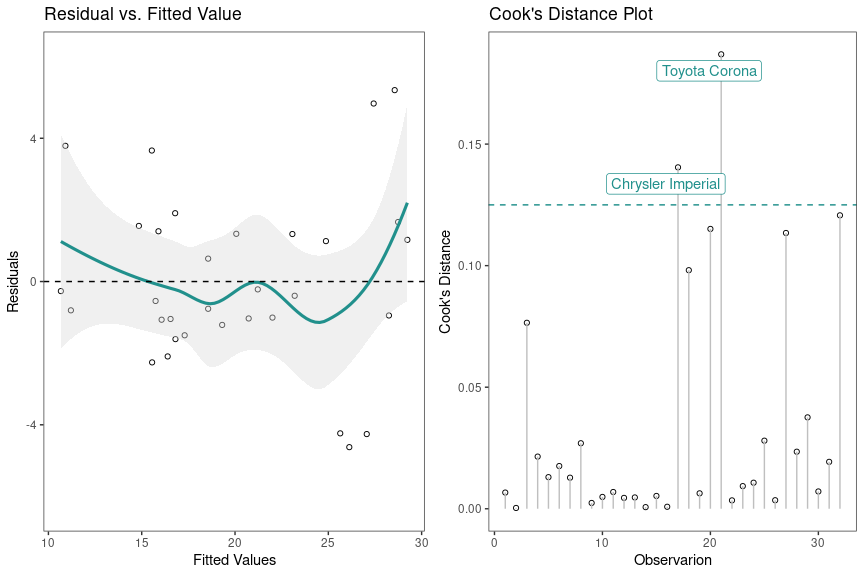## Half-Normal Plot

The Half-Normal plot is a graphical tool used to help identify which experiment factors have significant effects on the response. In addition, cutoff line(s) are added for the margin of error (ME) and the simultaneous margin of error (SME) of the effects. These values are obtained using unrepx::ME().

m1 <- lm(lns2 ~ (A+B+C+D)^4,data=original_epitaxial)
half_normal(m1)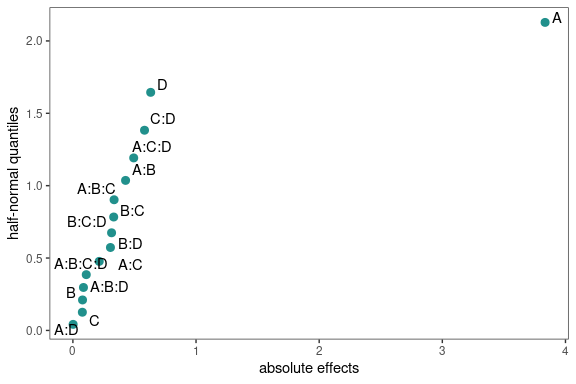With the method argument there are seven construction methods to obtain the pseudo standard errors in order to calculate (ME) and (SME). These methods are “Daniel”, “Dong”, “JuanPena”, “Lenth” (Default), “RMS”, “SMedian”, “Zahn”, “WZahn”. See ?ggDoE::half_normal for more details and references on each of the methods.

half_normal(m1,method='Daniel',alpha=0.1,
ref_line=TRUE,label_active=TRUE,
margin_errors=TRUE)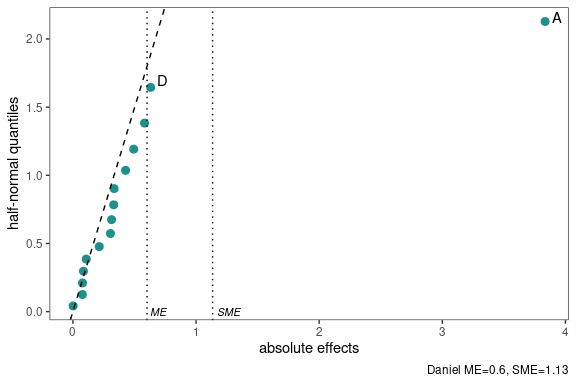You can change the significance level used to obtain (ME) and (SME) and determine which factors are active using the alpha argument. Default is alpha=0.05. Using label_active argument will only label the active factors in the experiment.

Using showplot=FALSE will return the needed information to reproduce/change the above half-normal plot

half_normal(m1,method='Daniel',alpha=0.1,
showplot=FALSE)
#> # A tibble: 15 × 3
#>    effects absolute_effects half_normal_quantiles
#>    <chr>              <dbl>                 <dbl>
#>  1 A:D              0.00197                0.0418
#>  2 C                0.0768                 0.126
#>  3 B                0.0783                 0.210
#>  4 A:B:D            0.0858                 0.297
#>  5 A:B:C:D          0.109                  0.385
#>  6 A:C              0.214                  0.477
#>  7 B:D              0.305                  0.573
#>  8 B:C:D            0.314                  0.674
#>  9 B:C              0.331                  0.784
#> 10 A:B:C            0.335                  0.903
#> 11 A:B              0.428                  1.04
#> 12 A:C:D            0.494                  1.19
#> 13 C:D              0.582                  1.38
#> 14 D                0.632                  1.64
#> 15 A                3.83                   2.13

## Interaction Effects Plot for a Factorial Design

Interaction effects plot between two factors in a factorial design

interaction_effects(adapted_epitaxial,response = 'ybar',
exclude_vars = c('s2','lns2'))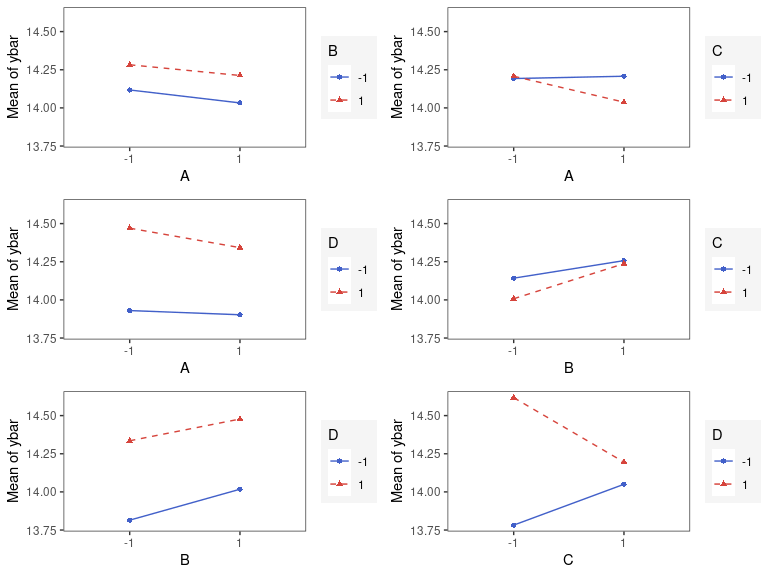interaction_effects(adapted_epitaxial,response = 'ybar',
exclude_vars = c('A','s2','lns2'),
n_columns=3)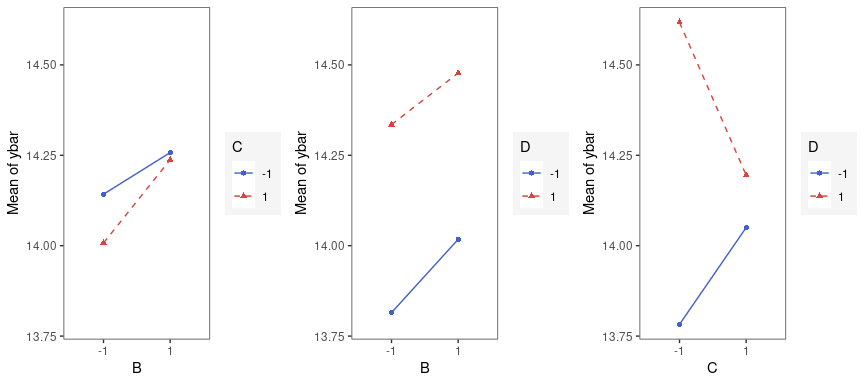## Main Effects Plot for a Factorial Design

Main effect plots for each factor in a factorial design

main_effects(original_epitaxial,
response='s2',
exclude_vars = c('ybar','lns2'))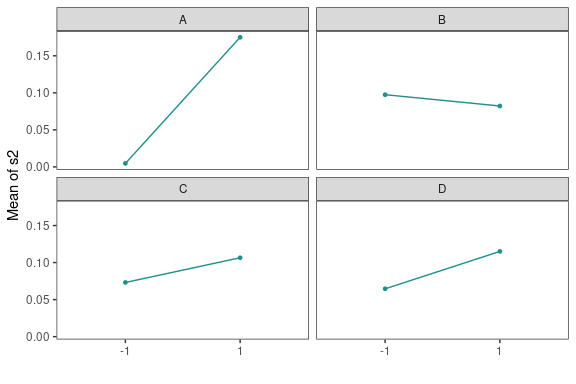main_effects(original_epitaxial,
response='s2',
exclude_vars = c('A','ybar','lns2'),
color_palette = 'viridis',
n_columns=3)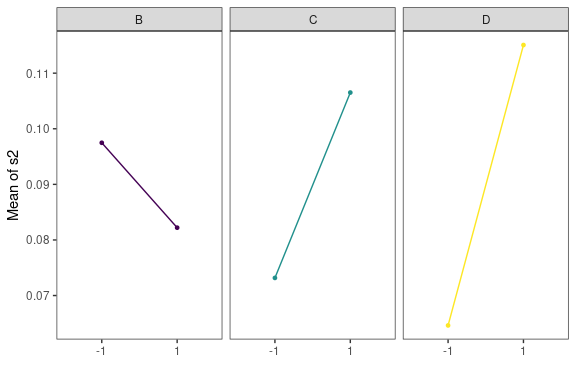## Contour Plots for Response Surface Methodology

heli.rsm <- rsm::rsm(ave ~ SO(x1, x2, x3, x4),
data = rsm::heli)

Using gg_rsm we can obtain contour plots that display the fitted surface for an rsm object. It is best to use rsm object such as the one defined above, as the plots below are produced using information from an rsm object. gg_rsm includes an ... argument for additional arguments from rsm::contour.lm, see rsm::contour.lm for more details on its arguments.

By default the contour plots produce will be in black and white color

gg_rsm(heli.rsm,form = ~x1+x2+x3,
at = rsm::xs(heli.rsm),
n_columns=2)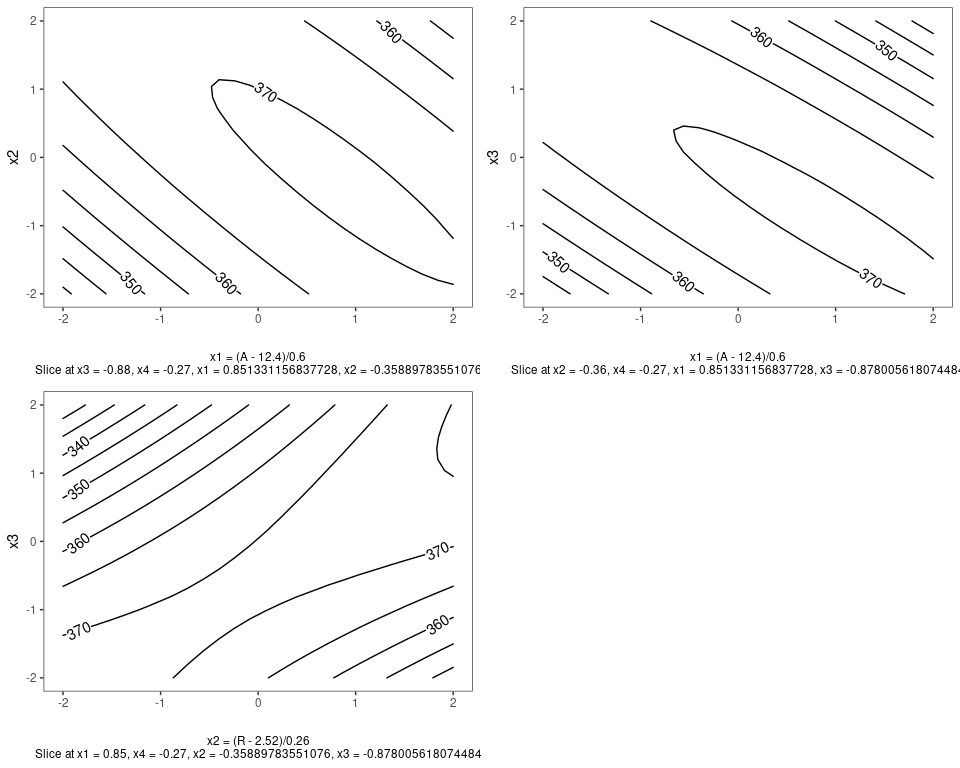with filled=TRUE, a viridis color scheme is applied.

gg_rsm(heli.rsm,form = ~x1+x2+x3,
at = rsm::xs(heli.rsm),
filled = TRUE,
n_columns=2)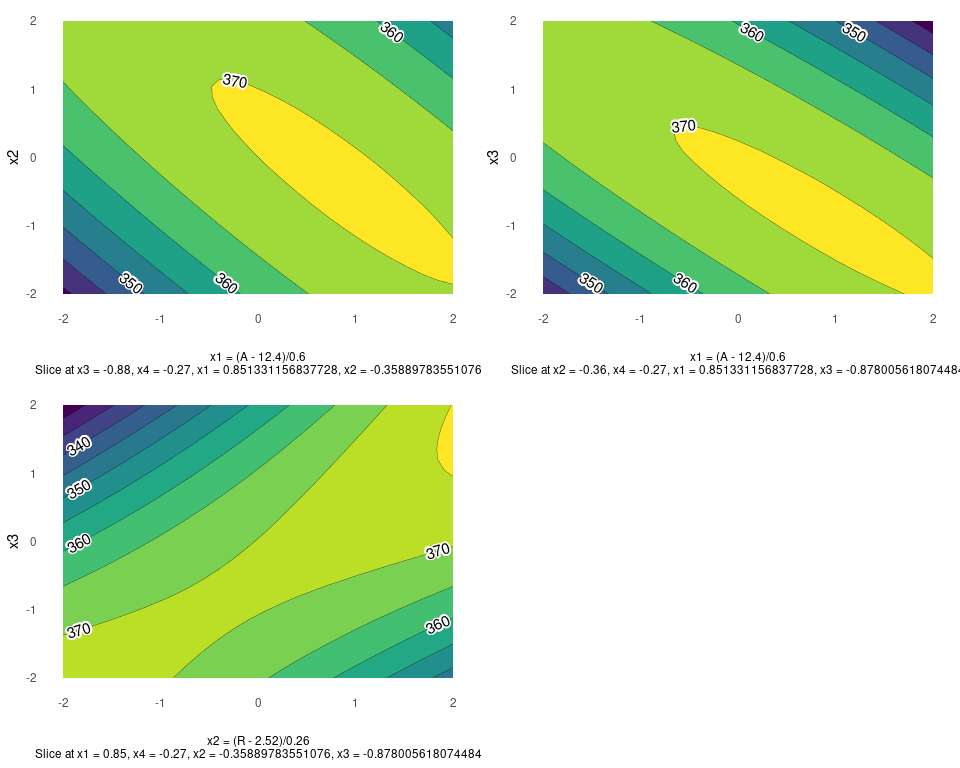## Pareto Plot

Pareto plot is a bar graph with the bars ordered by the size of the effect. In addition, cutoff line(s) are added for the margin of error (ME) and the simultaneous margin of error (SME) of the effects.

m1 <- lm(lns2 ~ (A+B+C+D)^4,data=original_epitaxial)
pareto_plot(m1)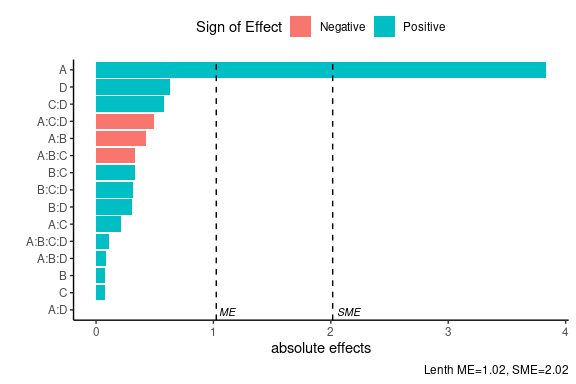As in the half_normal() function there are seven methods to compute the pseudo standard errors. For example, we consider ‘Daniel’ construction method using a significance level of $$\alpha=0.1$$. Default arguments for method is “Lenth” and alpha = 0.05.

pareto_plot(m1,method='Daniel',alpha=0.1)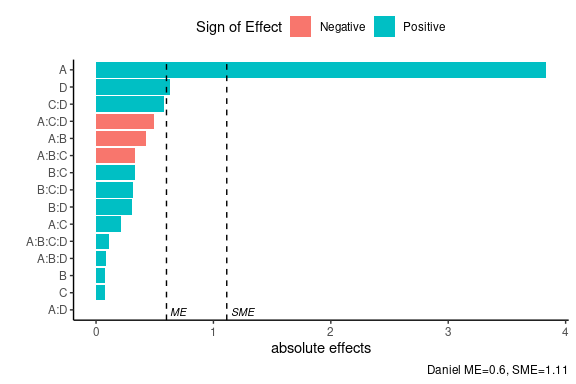If one uses the showplot=FALSE argument, a list will be returned with the calculated PSE,ME,SME as well as the data used to construct the pareto plot.

pareto_plot(m1,method='Daniel',
alpha=0.1, showplot=FALSE)
#> $errors #> # A tibble: 1 × 4 #> alpha PSE ME SME #> <dbl> <dbl> <dbl> <dbl> #> 1 0.1 0.335 0.600 1.11 #> #>$dat
#> # A tibble: 15 × 3
#>    effect_names  effects abs_effects
#>    <fct>           <dbl>       <dbl>
#>  1 A:D           0.00197     0.00197
#>  2 C             0.0768      0.0768
#>  3 B             0.0783      0.0783
#>  4 A:B:D         0.0858      0.0858
#>  5 A:B:C:D       0.109       0.109
#>  6 A:C           0.214       0.214
#>  7 B:D           0.305       0.305
#>  8 B:C:D         0.314       0.314
#>  9 B:C           0.331       0.331
#> 10 A:B:C        -0.335       0.335
#> 11 A:B          -0.428       0.428
#> 12 A:C:D        -0.494       0.494
#> 13 C:D           0.582       0.582
#> 14 D             0.632       0.632
#> 15 A             3.83        3.83

## Two Dimensional Projections

This function will output all two dimensional projections from a Latin hypercube design.

Below is an example from generating a random Latin hypercube design using the R package lhs

set.seed(10)
random_LHS <- lhs::randomLHS(n=15, k=4)
twoD_projections(random_LHS,n_columns=3,grid = TRUE)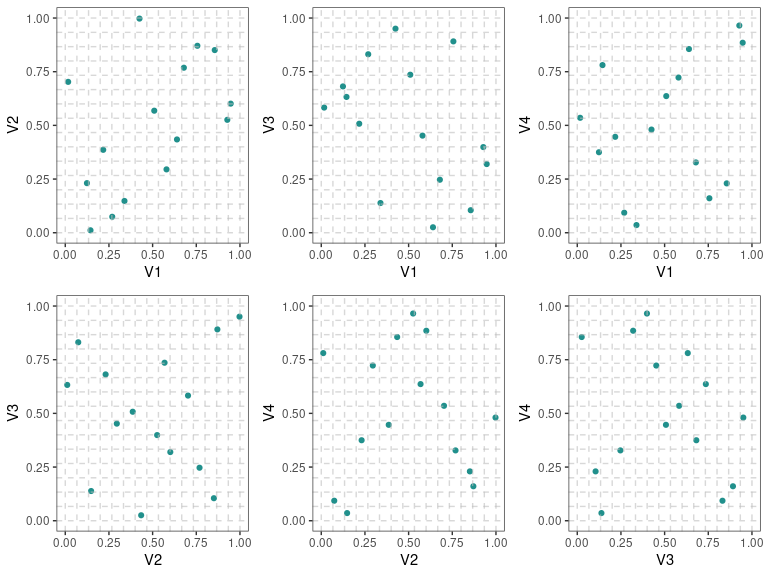Below is an example of a maximin Latin hypercube design with red color points and no grid (default setting)

maximin_LHS <- lhs::maximinLHS(n=15, k=4)
twoD_projections(maximin_LHS,n_columns=3,point_color = 'red')